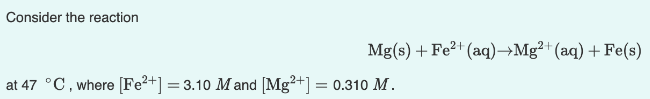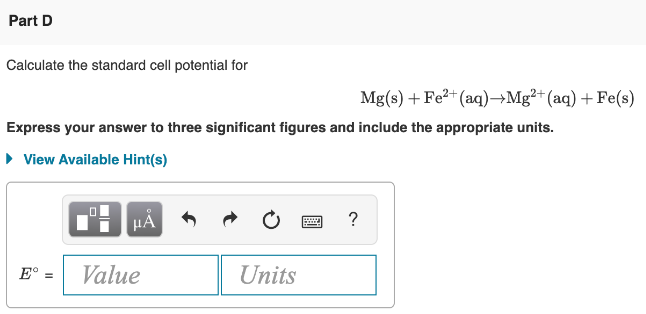# Consider the reaction Mg(s) + Fe^2+(aq) → Mg^2+(aq) + Fe(s) at 47°C, where [Fe^2+] = 3.10 M and [Mg^2+] = 0.310M Calculate the standard cell potential for Mg(s) + Fe^2+(aq) → Mg^2+(aq) + Fe(s) Express your answer to three significant figures and include the appropriate units.